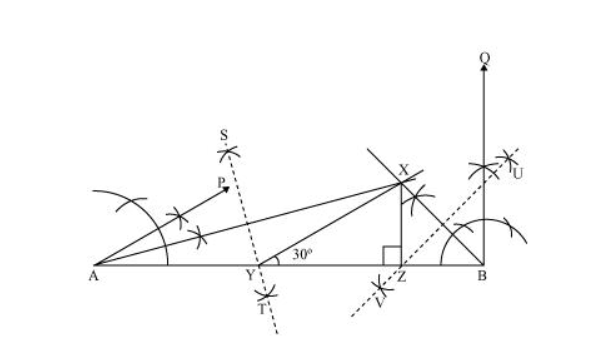# Construct a triangle XYZ in which ∠Y = 30°,

Question. Construct a triangle $X Y Z$ in which $\angle Y=30^{\circ}, \angle Z=90^{\circ}$ and $X Y+Y Z+Z X=11 \mathrm{~cm}$.

Solution:

The below given steps will be followed to construct the required triangle.

Step I: Draw a line segment AB of 11 cm.

(As $X Y+Y Z+Z X=11 \mathrm{~cm}$ )

Step II: Construct an angle, ∠PAB, of 30° at point A and an angle, ∠QBA, of 90° at point B.

Step III: Bisect ∠PAB and ∠QBA. Let these bisectors intersect each other at point X.

Step IV: Draw perpendicular bisector ST of AX and UV of BX.

Step V: Let ST intersect AB at Y and UV intersect AB at Z.

Join XY, XZ.

$\triangle X Y Z$ is the required triangle.